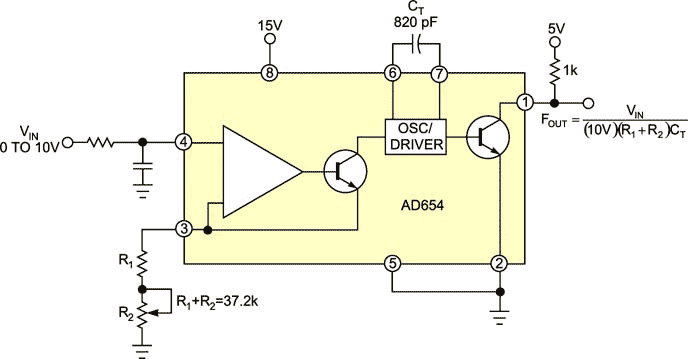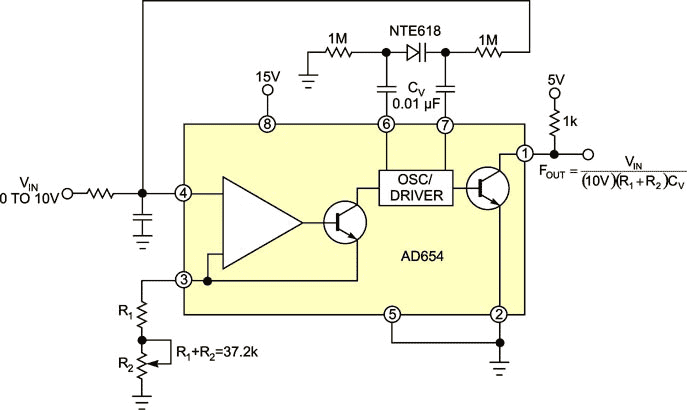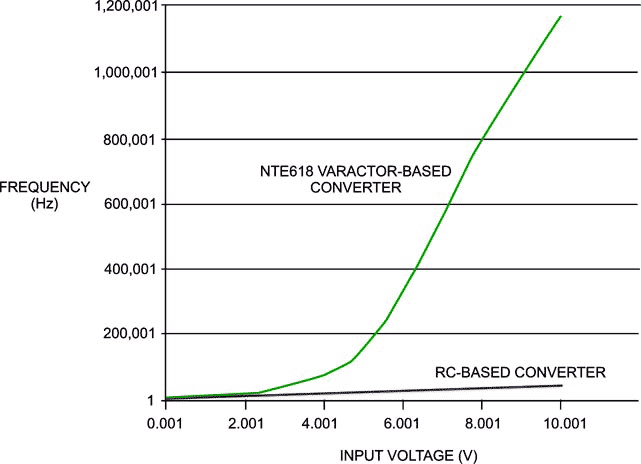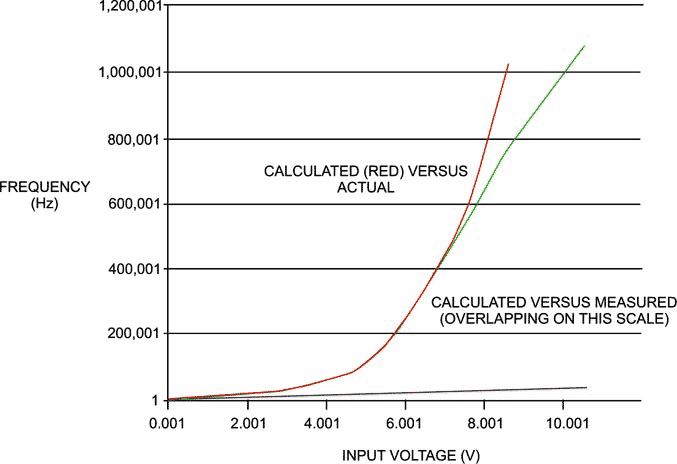# Dramatically increase the frequency range of RC-based voltage-controlled oscillators

Shawn Stafford, Pittsburgh, PA

EDN

A typical voltage-to-frequency converter – also known as a VCO (voltage-controlled oscillator) – IC has a simple linear tuning characteristic relating input voltage to output frequency. It is usually of the form F=kV/RC, where RC is the time constant of the associated timing resistor and capacitor. The output frequency range of these parts varies, but few, if any, tune over the entire range with one set of RC timing components. If you change the timing ratio as the input voltage changes, however, you can magnify the tuning range to take advantage of nearly the entire frequency range in one implementation.

One way to achieve this goal is to replace the timing capacitor with a variable capacitor whose capacitance inversely changes with bias voltage: a varactor diode (Reference 1). For this design, the Analog Devices AD654 voltage-to-frequency converter was considered because of its simplicity and bandwidth of at least 1 MHz (Reference 2).Figure 1. The usual usage of this VCO IC is with a fixed timing capacitor, CT, at pins 6 and 7.

Figure 1 shows a typical implementation using a fixed resistor and capacitor. For the values shown, this setup gives a frequency range of approximately 10 Hz to 30 kHz over 0 to 10V on the input. After replacing the timing capacitor with an NTE618 hyperabrupt varactor, as shown in Figure 2, the same input voltage range of 0 to 10V produced a tuning range of approximately 10 Hz to more than 1 MHz (Reference 3).Figure 2. A voltage-variable capacitor (varactor) and an ac-coupled dc-bias network replace the fixed timing capacitor.

The plot in Figure 3 compares the tuning curves of each converter configuration. Note the dramatic increase in range, but at the expense of linearity. Temperature stability will also be affected. Overall, precision is traded for tuning range, which should be acceptable in basic applications that do not require the specified precision.Figure 3. Replacing the fixed timing capacitor with a voltage-variable capacitor results in a much wider tuning range.

The hyperabrupt varactor allows large changes in frequency for small changes in bias voltage because of the large capacitance ratio. For some hyperabrupt varactors, the ratio can be as high as 15, as in the case of the NTE618, a varactor that AM receivers use. As the frequency of the converter increases with higher voltage, the capacitance decreases, which in turn increases the frequency. This combination of responses generates the wide tuning range. The 0.01-μF coupling capacitors separate the varactor bias voltage from the operation of the converter core. Light varactor biasing with high-value, 1M resistors prevents additional loading of the oscillator.

This behavior is calculable and predictable to some degree, even from the data sheets. The tuning curve for the varactor diode can be generated in Microsoft Excel. This information then can be used in the voltage-to-frequency conversion equation for the converter. For the NTE618, the approximate relationship of capacitance to voltage is expressed as

C = 800×10−10×e−0.46V.

Figure 4 shows the similarity between the calculated and measured results. The higher frequencies differ more as the varactor capacitance reduces to the order of the stray capacitance in the circuit and parts. Careful layout can minimize this issue and increase the range.Figure 4. The calculated and measured responses are in close agreement.

Note that at low input voltages, the varactor-based response and the fixed-capacitor converter response are nearly identical because of the varactor’s inverse exponential relationship to the voltage. One useful result of achieving this range is eliminating the need to switch between converters to extend the tuning range. You can explore other useful and interesting applications using this approach with phase-locked loops, modulators, or function generators.

Editor’s note:

Figure 13 of the Analog Devices AD654 data sheet contains errors (Reference 2). The 74LS86 and LM360 cannot be subjected to 15V, and R7 is most likely 8.2k, not 8.2Ω.

References

1. Williams, Jim, and David Beebe, “Switching-regulator supply provides low-noise biasing for varactor diodes,”EDN, Nov 9, 2000, pg 117.
2. A2“AD654: Low Cost MonolithicVoltage-to-Frequency Converter,” Analog Devices.
3. “NTE618 Varactor Silicon Tuning Diode for AMRadio,” NTE Electronics.

EDN